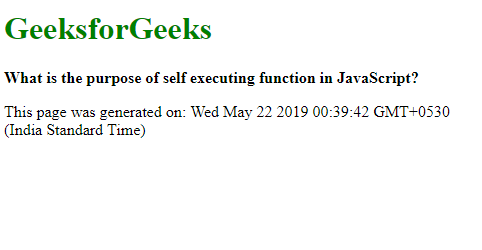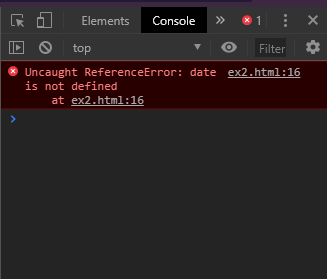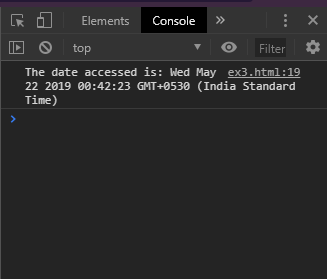Open in App
Not now

# What is the purpose of self executing function in JavaScript?

• Last Updated : 19 Jan, 2023

The self-executing anonymous function is a special function which is invoked right after it is defined. There is no need to call this function anywhere in the script. This type of function has no name and hence it is called an anonymous function. The function has a trailing set of parenthesis. The parameters for this function could be passed in the parenthesis.

Syntax:

```(function (parameters) {
// Function body
})(arguments);```

Example:

## html

 ```<``html``>` `<``head``>``    ``<``title``>``        ``What is the purpose of self``        ``executing function in JavaScript?``    ````` `<``body``>``    ``<``h1` `style="color: green">``        ``GeeksforGeeks``    ````    ` `    ``<``b``>``        ``What is the purpose of self``        ``executing function in JavaScript?``    ````    ` `    ``<``p``>``        ``This page was generated on:``        ``<``span` `class="output">``    ````    ` `    ``<``script` `type="text/javascript">``        ``(function () {``            ``date = new Date().toString();``            ` `            ``document.querySelector('.output').textContent``                        ``= date;``        ``})();``    ````` ``

Output:Why use an anonymous function?

The advantage of using an anonymous function rather than writing the code directly is that the variables and functions defined within the anonymous function are not available to the code outside it. This prevents the global namespace from becoming littered with variables and functions that may not be needed further. It can be also be used to enable access to only the variables and functions. This is shown in the below examples.

Accessing a variable from outside the anonymous function: This example shows that accessing the date object from outside the anonymous function results in an error.

## html

 ```<``html``>` `<``head``>``    ``<``title``>``        ``What is the purpose of self``        ``executing function in JavaScript?``    ````` `<``body``>``    ``<``h1` `style="color: green">``        ``GeeksforGeeks``    ````    ` `    ``<``b``>``        ``What is the purpose of self``        ``executing function in JavaScript?``    ````    ` `    ``<``p``>``        ``This page was generated on:``        ``<``span` `class="output">``    ````    ` `    ``<``script` `type="text/javascript">``        ``(function () {``            ``let date = new Date().toString();``            ` `            ``document.querySelector('.output').textContent``                    ``= date;``        ``})();` `        ``console.log('The date accessed is: ' + date);``    ````` `                   `

Output:Allowing access to one variable to outside the function: This example shows that the date variable could be made available outside the function by making it global.

## html

 ```<``html``>` `<``head``>``    ``<``title``>``        ``What is the purpose of self``        ``executing function in JavaScript?``    ````` `<``body``>``    ``<``h1` `style="color: green">``        ``GeeksforGeeks``    ````    ` `    ``<``b``>``        ``What is the purpose of self``        ``executing function in JavaScript?``    ````    ` `    ``<``p``>``        ``This page was generated on:``        ``<``span` `class="output">``    ````    ` `    ``<``script` `type="text/javascript">``        ``(function () {``            ``let date = new Date().toString();``            ``document.querySelector('.output').textContent = date;``    ` `            ``// Assign to global window making it``            ``// accessible to outside``            ``window.date = date;``        ``})();``    ` `        ``console.log('The date accessed is: ' + date);``    ````` `                   `

Output:My Personal Notes arrow_drop_up